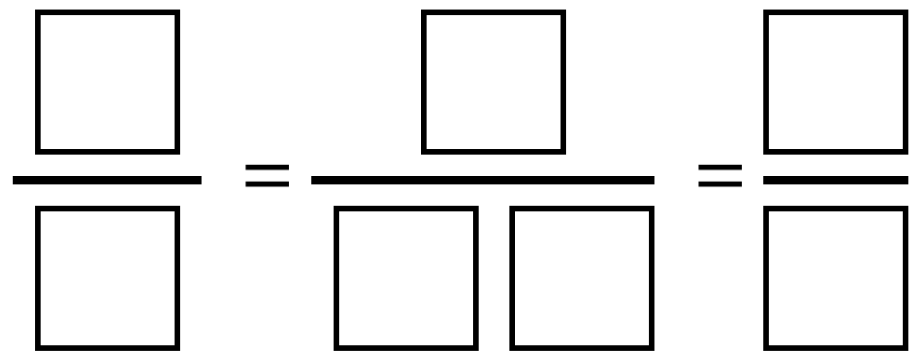Home > Grade 4 > Finding Equivalent Fractions

# Finding Equivalent Fractions

Directions: Using the digits 1 to 9 at most one time each, fill in the boxes to create 3 equivalent fractions.### Hint

Think benchmark fractions.

2/4 = 3/6 = 9/18
1/3 = nope
1/4 = 2/8 = 9/36 yay
2/3 = nope
3/4 = 6/8 = 9/12 yay

Source: Graham FletcherBowen Kerins

## Multiplying Two-Digit Numbers – Closest to 7,000

Directions: Directions: Using the digits 1 to 9 at most one time each, fill in …

1.Am I correct that the two 1/2 options with 5/10 would not work based on the instructions?

•Hi Jenny,
You’re correct based on the directions because 10 contains a zero. An equivalence of half could be used, just not if it’s expressed as 1/2.

•I was just looking at that – I think the instructions should say 0 through 9. I was also wondering about 1/2=3/6=4/8, there is no two digit denominator unless a student uses zero in the tens place.

•Robert Kaplinsky

Thanks Tamara. I updated the answer to remove the one that had a 0 in it.

2.I changed this to increase the amount of digits. This actually created less answers but with it be a follow up, students have to engage in even more strategic thinking (applying what they did on this problem)

It was blank/blank = blank blank / blank blank = blank / blank blank

One solution thus far is 3/6 = 29/58 = 7/14

I am sure there are more though.

•My students did this today and came up with two creative solutions.

One student came up with 3/6=4.5/9= 1/2. This student added the decimal between the 4 and 5 to make it true.

The other student flipped the equation upside down and came up with fractions greater than one. 6/3=18/9=4/2